Courses

# Indefinite Integrals Mathematics Notes | EduRev

## Mathematics : Indefinite Integrals Mathematics Notes | EduRev

The document Indefinite Integrals Mathematics Notes | EduRev is a part of the Mathematics Course Calculus for IIT JAM Mathematics.
All you need of Mathematics at this link: Mathematics

Indefinite Integrals
In the past two chapters we’ve been given a function, f(x), and asking what the derivative of this function was. Starting with this section we are now going to turn things around. We now want to ask what function we differentiated to get the function f(x).
Let’s take a quick look at an example to get us started.
Example 1: What function did we differentiate to get the following function.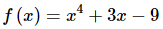Solution: Let’s actually start by getting the derivative of this function to help us see how we’re going to have to approach this problem. The derivative of this function is,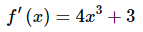The point of this was to remind us of how differentiation works. When differentiating powers of x we multiply the term by the original exponent and then drop the exponent by one.Now, let’s go back and work the problem. In fact, let’s just start with the first term. We got x4 by differentiating a function and since we drop the exponent by one it looks like we must have differentiated x5. However, if we had differentiated x5 we would have 5xand we don’t have a 5 in front our first term, so the 5 needs to cancel out after we’ve differentiated. It looks then like we would have to differentiate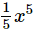in order to get x4.
Likewise, for the second term, in order to get 3x after differentiating we would have to differentiate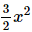Again, the fraction is there to cancel out the 2 we pick up in the differentiation.The third term is just a constant and we know that if we differentiate xx we get 1. So, it looks like we had to differentiate -9xx to get the last term.Putting all of this together gives the following function,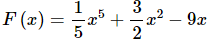Our answer is easy enough to check. Simply differentiate F(x).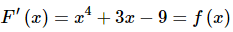So, it looks like we got the correct function. Or did we? We know that the derivative of a constant is zero and so any of the following will also give f(x) upon differentiating.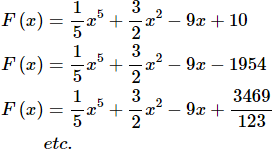In fact, any function of the form,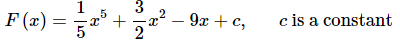will give f(x) upon differentiating.
There were two points to this last example. The first point was to get you thinking about how to do these problems. It is important initially to remember that we are really just asking what we differentiated to get the given function.
The other point is to recognize that there are actually an infinite number of functions that we could use and they will all differ by a constant.
Now that we’ve worked an example let’s get some of the definitions and terminology out of the way.

Definitions
Given a function, f(x), an anti-derivative of f(x) is any function F(x) such that
F′(x)=f(x)
If F(x) is any anti-derivative of f(x) then the most general anti-derivative of f(x) is called an indefinite integral and denoted,
∫ f(x)dx=F(x)+c, is any constant
In this definition the ∫is called the integral symbol, f(x) is called the integrand, x is called the integration variable and the “c” is called the constant of integration.
Note that often we will just say integral instead of indefinite integral (or definite integral for that matter when we get to those). It will be clear from the context of the problem that we are talking about an indefinite integral (or definite integral).
The process of finding the indefinite integral is called integration or integrating f(x).
If we need to be specific about the integration variable we will say that we are integrating f(x) with respect to x.
Let’s rework the first problem in light of the new terminology.

Example 2: Evaluate the following indefinite integral.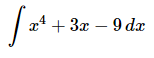Solution: Since this is really asking for the most general anti-derivative we just need to reuse the final answer from the first example.
The indefinite integral is,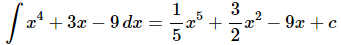A couple of warnings are now in order. One of the more common mistakes that students make with integrals (both indefinite and definite) is to drop the dx at the end of the integral. This is required! Think of the integral sign and the dx as a set of parentheses. You already know and are probably quite comfortable with the idea that every time you open a parenthesis you must close it. With integrals, think of the integral sign as an “open parenthesis” and the dx as a “close parenthesis”.
If you drop the dx it won’t be clear where the integrand ends. Consider the following variations of the above example.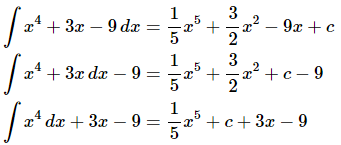You only integrate what is between the integral sign and the dx. Each of the above integrals end in a different place and so we get different answers because we integrate a different number of terms each time. In the second integral the “-9” is outside the integral and so is left alone and not integrated. Likewise, in the third integral the “3x−93x−9” is outside the integral and so is left alone.Knowing which terms to integrate is not the only reason for writing the dxdx down. In the Substitution Rule section we will actually be working with the dxdx in the problem and if we aren’t in the habit of writing it down it will be easy to forget about it and then we will get the wrong answer at that stage.The moral of this is to make sure and put in the dxdx! At this stage it may seem like a silly thing to do, but it just needs to be there, if for no other reason than knowing where the integral stops.On a side note, the dxdx notation should seem a little familiar to you. We saw things like this a couple of sections ago. We called the dxdx a differential in that section and yes that is exactly what it is. The dxdx that ends the integral is nothing more than a differential.The next topic that we should discuss here is the integration variable used in the integral. Actually, there isn’t really a lot to discuss here other than to note that the integration variable doesn’t really matter. For instance,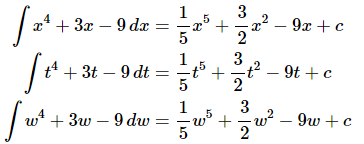Changing the integration variable in the integral simply changes the variable in the answer. It is important to notice however that when we change the integration variable in the integral we also changed the differential (dx, dt, or dw) to match the new variable. This is more important than we might realize at this point.Another use of the differential at the end of integral is to tell us what variable we are integrating with respect to. At this stage that may seem unimportant since most of the integrals that we’re going to be working with here will only involve a single variable. However, if you are on a degree track that will take you into multi-variable calculus this will be very important at that stage since there will be more than one variable in the problem. You need to get into the habit of writing the correct differential at the end of the integral so when it becomes important in those classes you will already be in the habit of writing it down.To see why this is important take a look at the following two integrals.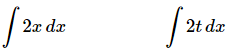The first integral is simple enough.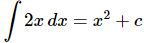The second integral is also fairly simple, but we need to be careful. The dx tells us that we are integrating x’s. That means that we only integrate x’s that are in the integrand and all other variables in the integrand are considered to be constants. The second integral is then,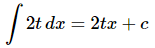So, it may seem silly to always put in the dx, but it is a vital bit of notation that can cause us to get the incorrect answer if we neglect to put it in.
Now, there are some important properties of integrals that we should take a look at.
1. ∫ k f (x) dx= k ∫ f(x) dx where k is any number. So, we can factor multiplicative constants out of indefinite integrals.
2. ∫−f (x) dx=−∫ f(x) dx. This is really the first property with k=−1 and so no proof of this property will be given.
3. ∫ f (x) ± g (x) dx =∫ f(x) dx ± ∫ g (x) dx. In other words, the integral of a sum or difference of functions is the sum or difference of the individual integrals. This rule can be extended to as many functions as we need.
Notice that when we worked the first example above we used the first and third property in the discussion. We integrated each term individually, put any constants back in and then put everything back together with the appropriate sign.
Not listed in the properties above were integrals of products and quotients. The reason for this is simple. Just like with derivatives each of the following will NOT work.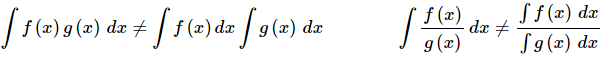With derivatives we had a product rule and a quotient rule to deal with these cases. However, with integrals there are no such rules. When faced with a product and quotient in an integral we will have a variety of ways of dealing with it depending on just what the integrand is.There is one final topic to be discussed briefly in this section. On occasion we will be given f′(x) and will ask what f(x) was. We can now answer this question easily with an indefinite integral.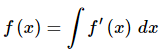Example 3: If f′ (x) =x+ 3x−9 what was f(x)?
Solution: By this point in this section this is a simple question to answer.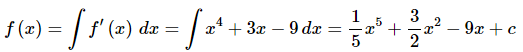In this section we kept evaluating the same indefinite integral in all of our examples. The point of this section was not to do indefinite integrals, but instead to get us familiar with the notation and some of the basic ideas and properties of indefinite integrals. The next couple of sections are devoted to actually evaluating indefinite integrals.

Practice problems: Infinite integrals
Q.1. Evaluate each of the following indefinite integrals.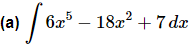Ans. All we are being asked to do here is “undo” a differentiation and if you recall the basic differentiation rules for polynomials this shouldn’t be too difficult. As we saw in the notes for this section all we really need to do is increase the exponent by one (so upon differentiation we get the correct exponent) and then fix up the coefficient to make sure that we will get the correct coefficient upon differentiation.
Here is the answer for this part.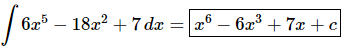Don’t forget the “+c”! Remember that the original function may have had a constant on it and the “+c” is there to remind us of that.
Also, don’t forget that you can easily check your answer by differentiating your answer and making sure that the result is the same as the integrand.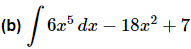Ans. This part is not really all that different from the first part. The only difference is the placement of the dx. Recall that one of the things that the dx tells us where to end the integration. So, in the part we are only going to integrate the first term.
Here is the answer for this part.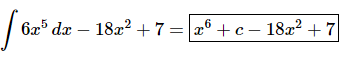Q.2. Evaluate each of the following indefinite integrals.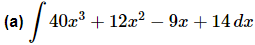Ans. All we are being asked to do here is “undo” a differentiation and if you recall the basic differentiation rules for polynomials this shouldn’t be too difficult. As we saw in the notes for this section all we really need to do is increase the exponent by one (so upon differentiation we get the correct exponent) and then fix up the coefficient to make sure that we will get the correct coefficient upon differentiation.
Here is that answer for this part.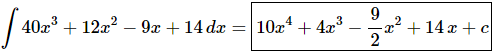Don’t forget the “+c”! Remember that the original function may have had a constant on it and the “+c” is there to remind us of that.
Also, don’t forget that you can easily check your answer by differentiating your answer and making sure that the result is the same as the integrand.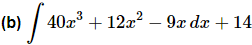Ans. This part is not really all that different from the first part. The only difference is the placement of the dx. Recall that one of the things that the dx tells us where to end the integration. So, in the part we are only going to integrate the first term.
Here is the answer for this part.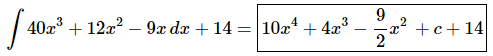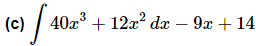Ans. The only difference between this part and the previous part is that the location of the dx moved.
Here is the answer for this part.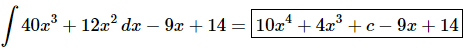For problems 3 – 5 evaluate the indefinite integral.
Q.3. Evaluate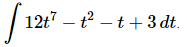Ans. All we are being asked to do here is “undo” a differentiation and if you recall the basic differentiation rules for polynomials this shouldn’t be too difficult. As we saw in the notes for this section all we really need to do is increase the exponent by one (so upon differentiation we get the correct exponent) and then fix up the coefficient to make sure that we will get the correct coefficient upon differentiation.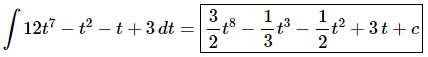Don’t forget the “+c”! Remember that the original function may have had a constant on it and the “+c” is there to remind us of that.
Also, don’t forget that you can easily check your answer by differentiating your answer and making sure that the result is the same as the integrand.

Q.4. Evaluate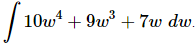Ans. All we are being asked to do here is “undo” a differentiation and if you recall the basic differentiation rules for polynomials this shouldn’t be too difficult. As we saw in the notes for this section all we really need to do is increase the exponent by one (so upon differentiation we get the correct exponent) and then fix up the coefficient to make sure that we will get the correct coefficient upon differentiation.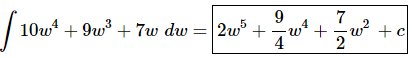Don’t forget the “+c”! Remember that the original function may have had a constant on it and the “+c” is there to remind us of that.
Also, don’t forget that you can easily check your answer by differentiating your answer and making sure that the result is the same as the integrand.

Q.5. Evaluate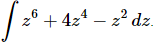Ans. All we are being asked to do here is “undo” a differentiation and if you recall the basic differentiation rules for polynomials this shouldn’t be too difficult. As we saw in the notes for this section all we really need to do is increase the exponent by one (so upon differentiation we get the correct exponent) and then fix up the coefficient to make sure that we will get the correct coefficient upon differentiation.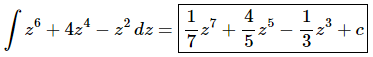Don’t forget the “+c”! Remember that the original function may have had a constant on it and the “+c” is there to remind us of that.
Also, don’t forget that you can easily check your answer by differentiating your answer and making sure that the result is the same as the integrand.

Q.6. Determine f(x) given that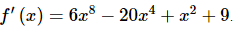Ans. We know that indefinite integrals are asking us to undo a differentiation to so all we are really being asked to do here is evaluate the following indefinite integral.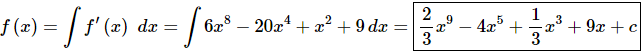Don’t forget the “+c”! Remember that the original function may have had a constant on it and the “+c” is there to remind us of that.

Q.7. Determine h(t) given that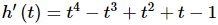Ans. We know that indefinite integrals are asking us to undo a differentiation to so all we are really being asked to do here is evaluate the following indefinite integral.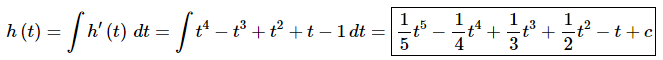Don’t forget the “+c”! Remember that the original function may have had a constant on it and the “+c” is there to remind us of that.

Offer running on EduRev: Apply code STAYHOME200 to get INR 200 off on our premium plan EduRev Infinity!

,

,

,

,

,

,

,

,

,

,

,

,

,

,

,

,

,

,

,

,

,

;